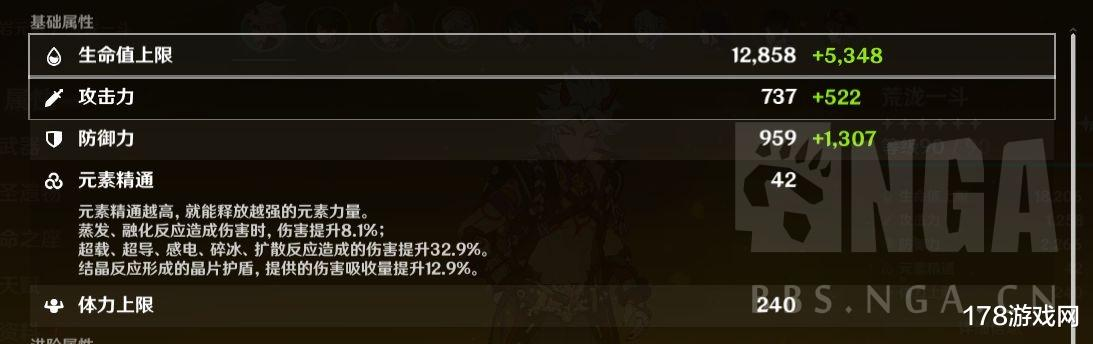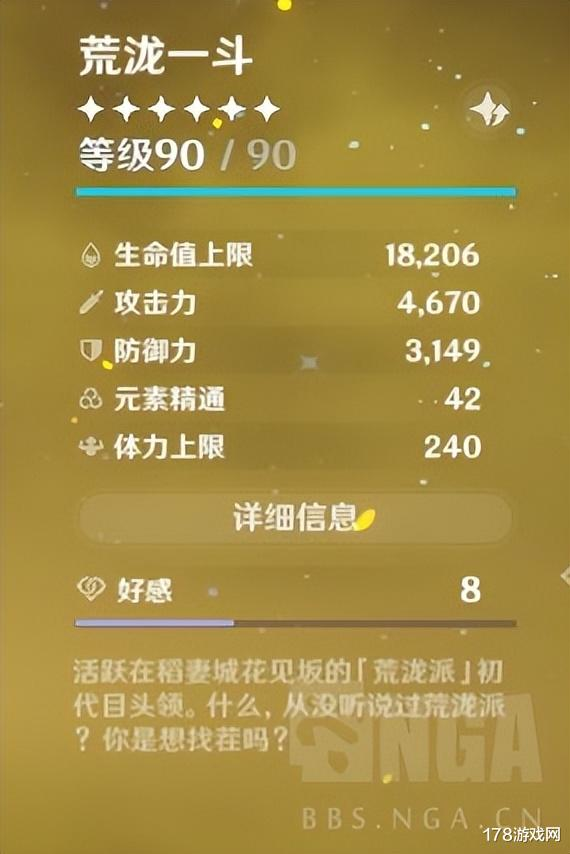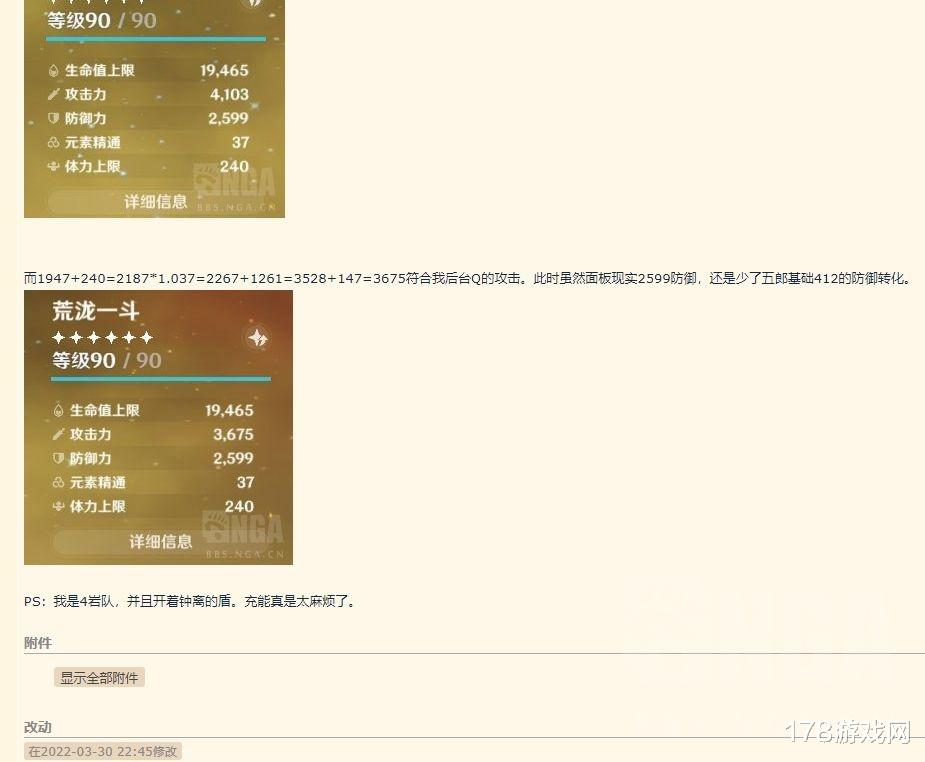# 原神：关于一斗alt直接切大招吃不到五郎buff解决办法959*0.25=239.75

737*0.2=147.4

959*0.24=230.16

959*0.18=172.62

959*0.12=115.08

959*0.06=57.54

959+1307+240+412+231=3149与我第一个视频开完大招后触发4层华馆的防御相同。

3149*1.037=3265.513

3265+1258=4523

4523+147(五郎宗室)=4670正好与开完大招后截图相同。BV1F34y1L7VP